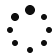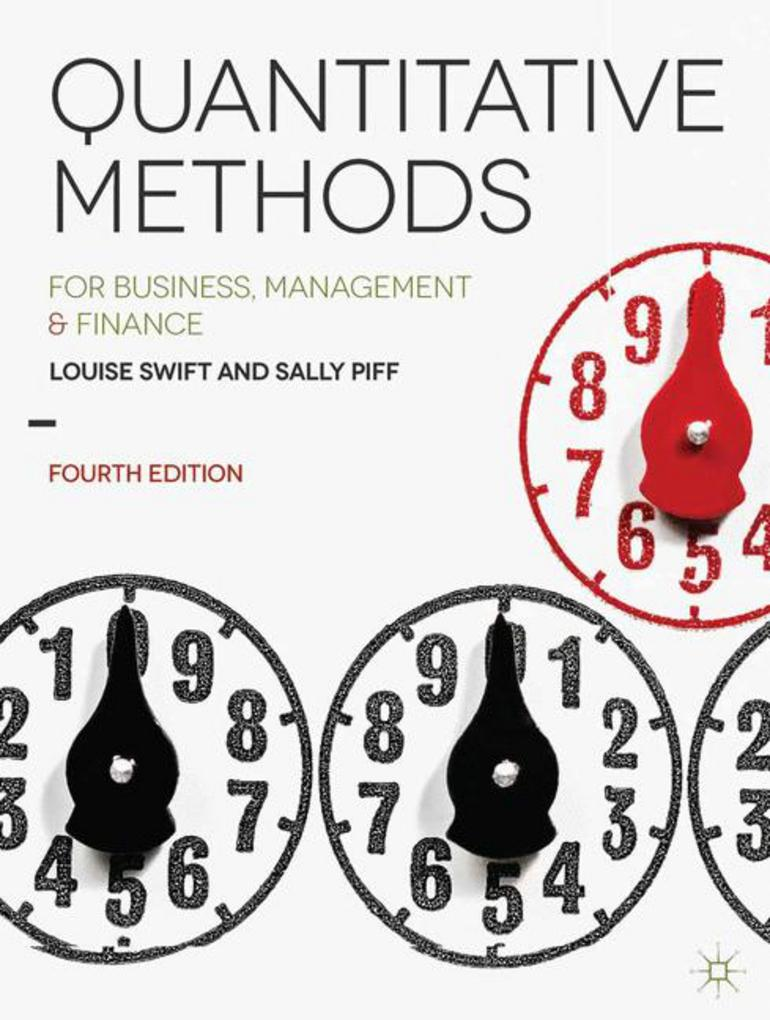### PART I: ESSENTIAL MATHS

1. Numbers and Symbols

2. Simplifying Expressions

3. Solving Problems

4. Modelling Using Straight Lines

### PART II: MORE MATHS

5. Some Special Equations

6. Modelling Using Curves

7. Rates of Change

PART III: DESCRIBING DATA

8. Pictures of Data

9. Summarising Data

### PART IV: PROBABILITY

10. Measuring Uncertainty

11. Numerical Outcomes

12. Continuous Numerical Outcomes

13. Some More Probability Distributions

### PART V: STATISTICS

14. Estimation

15. Testing Hypotheses

16. Correlation and Regression

17. Comparing Two Populations

18. Nonparametric methods

20. Forecasting

21. Statistics in Practice

22. Linear Programming Models

23. Planning Projects

24. Models for Inventory Control

25. Time and Money

26. Decision Making

27. Simulating Reality

28. Controlling Quality

PART VII: STATISTICAL TABLES

29. Cumulative Binomial Probabilities

30. Cumulative Standard Normal Probabilities

31. Percentage Points of the Standard Normal Distribution

32. Random Digits

33. Percentage Points of the t Distribution

34. Percentage Points of the x2 Distribution.